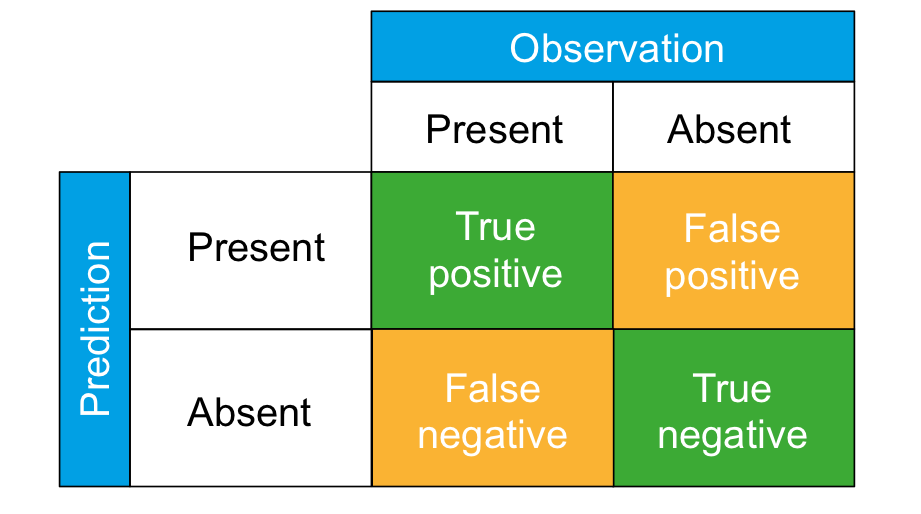A vital step in modelling is assessing the accuracy of the model prediction, commonly called ‘validation’ or ‘evaluation’. While most SDM modelling methods are designed to calculate a probability of species occurrence in a particular place, these probabilistic predictions are then converted to a categorical prediction (presence/absence) based on a threshold probability value.

The categorical predictions of an SDM model can either match the observed presences (true positive) and absences (true negative) of a species, or the model predicts a presence or absence that is not consistent with the observations. There are two different types of prediction errors that can be made: a ‘false positive’ occurs when the model predicts a species to be present in places where it has not been observed, whereas a ‘false negative’ means that the model predicted a species to be absent in places where it is observed to be present. The performance of a model can be summarized in a so-called contingency table or confusion matrix as shown below.A perfect model would only produce ‘true positives’ and ‘true negatives’, and not include any ‘false positives’ or ‘false negatives’. The elements of the contingency table are used to compute various evaluation criteria that measure the performance of the model.